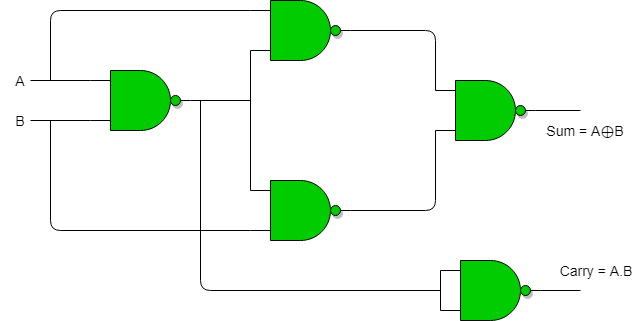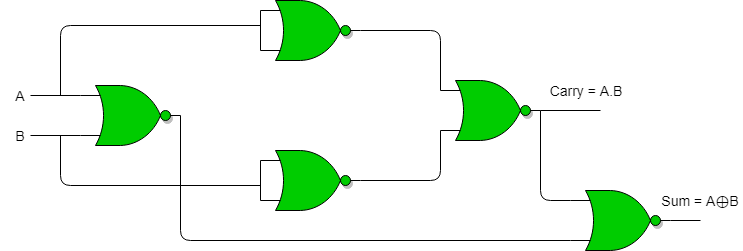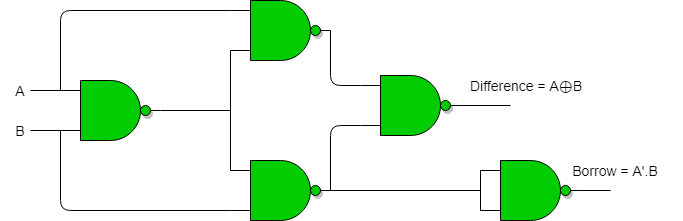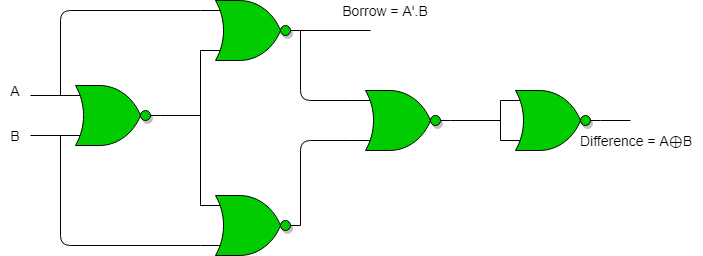Open In App

# Half Adder and Half Subtractor using NAND NOR gates

### Advantages of using NAND and NOR gates to implement Half Adder and Half Subtractor:

1. Universality: NAND and NOR gates are considered universal gates because they can be used to implement any logical function, including binary arithmetic functions such as addition and subtraction.
2. Cost-effectiveness: NAND and NOR gates are relatively simple and inexpensive to manufacture compared to other types of gates.
3. Reduced power consumption: NAND and NOR gates consume less power compared to other types of gates, making them suitable for low-power applications.

### Disadvantages of using NAND and NOR gates to implement Half Adder and Half Subtractor:

1. Propagation delay: The propagation delay of NAND and NOR gates can be higher compared to other types of gates, which can affect the overall performance of the system.
2. Noise susceptibility: NAND and NOR gates can be susceptible to noise and other types of interference, which can cause incorrect operation of the circuit.
3. In conclusion, while NAND and NOR gates have their advantages, they are not suitable for all applications. The choice of gates depends on the specific requirements of the circuit and the design trade-offs between performance, cost, and power consumption.

Implementation of Half Adder using NAND gates : Total 5 NAND gates are required to implement half adder.Implementation of Half Adder using NOR gates : Total 5 NOR gates are required to implement half adder.Implementation of Half Subtractor using NAND gates : Total 5 NAND gates are required to implement half subtractor.Implementation of Half Subtractor using NOR gates : Total 5 NOR gates are required to implement half subtractor.This article is contributed by Sumouli Choudhury